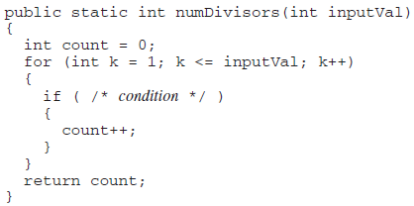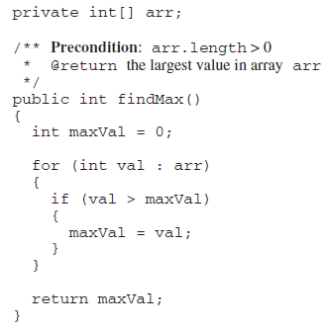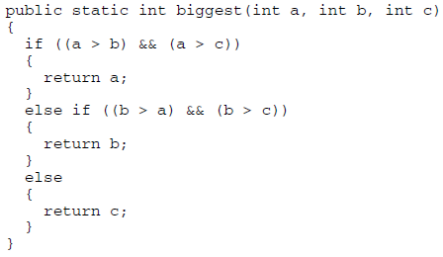# 2015 AP Computer Science A 计算机科学A真题系列之Released Exam选择题免费下载

### 真题与答案下载### 2015 AP 计算机科学A公开卷选择题部分免费下载

#### 部分真题预览：

1）Consider the following incomplete method, which is intended to return the number of integers that evenly divide the integer inputVal. Assume that inputVal is greater than 0.Which of the following can be used to replace / * condition * / so that numDivisors will work as intended?

1. inputVal % k == 0
2. k % inputVal == 0
3. inputVal % k != 0
4. inputVal / k == 0
5. k / inputVal > 0

4)Consider the following instance variable and method.Method findMax is intended to return the largest value in the array arr. Which of the following best describes the conditions under which the method findMax will not work as intended?

1. The largest value in arr occurs only once and is in arr .
2. The largest value in arr occurs only once and is in arr[arr.length - 1].
3. The largest value in arr is negative.
4. The largest value in arr is zero.
The largest value in arr occurs more than once.

14)Consider the following method, biggest, which is intended to return the greatest of three integers. It does not always work as intended.Which of the following best describes the error in the method?

1. biggest always returns the value of a.
2. biggest may not work correctly when c has the greatest value.
3. biggest may not work correctly when a and b have equal values.
4. biggest may not work correctly when a and c have equal values.
5. biggest may not work correctly when b and c have equal values.